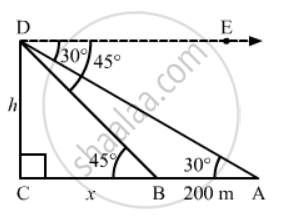Share

# The Angles of Depression of Two Ships from the Top of a Light House and on the Same Side of It Are Found to Be 45° and 30° Respectively. If the Ships Are 200 M Apart, Find the Height of the Light - CBSE Class 10 - Mathematics

#### Question

The angles of depression of two ships from the top of a lighthouse and on the same side of it are found to be 45° and 30° respectively. If the ships are 200 m apart, find the height of the lighthouse.

#### SolutionLet CD be the light house and A and B be the positions of the two ships.

AB = 200 m    (Given)

Suppose CD = h m and BC = m

Now,

∠DAC = ∠ADE = 30º        (Alternate angles)

∠DBC =∠EDB = 45º        (Alternate angles)

In right ∆BCD,

tan 45^@ = (CD)/(BC)

=> 1 = h/x

=> x = h .....(1)

In right ∆ACD,

tan 30^@ = (CD)/(AC)

=> 1/sqrt3 = h/(x + 200)

 => sqrt3h = x + 200    ......(2)

From (1) and (2), we get

sqrt3h = 200 + h

=> sqrt3h - h = 200

=> (sqrt3 - 1)h = 200

=> h = 200/(sqrt3 - 1)

=> h = (200(sqrt3 + 1))/(((sqrt3 -1)(sqrt3 + 1))

=> h = (200(sqrt3 + 1))/2 = 100(sqrt3 + 1)m

Hence, the height of the light house is 100(sqrt3 + 1)` m

Is there an error in this question or solution?

#### Video TutorialsVIEW ALL 

Solution The Angles of Depression of Two Ships from the Top of a Light House and on the Same Side of It Are Found to Be 45° and 30° Respectively. If the Ships Are 200 M Apart, Find the Height of the Light Concept: Heights and Distances.
S